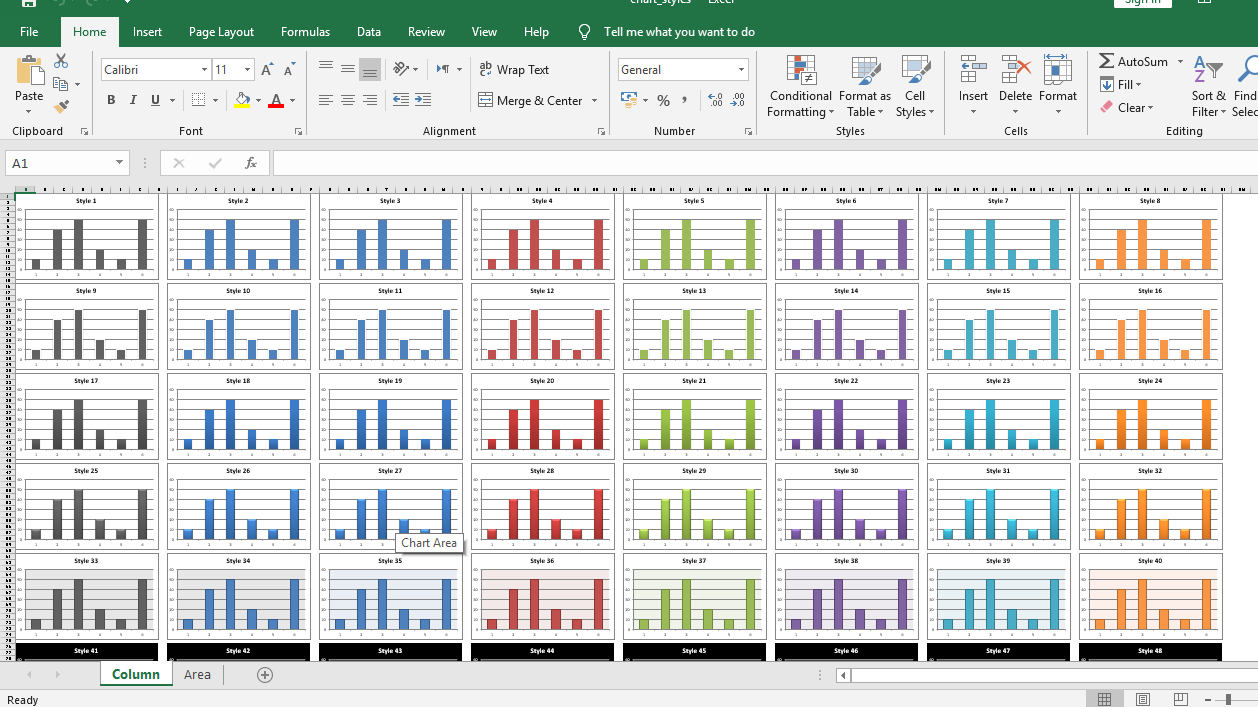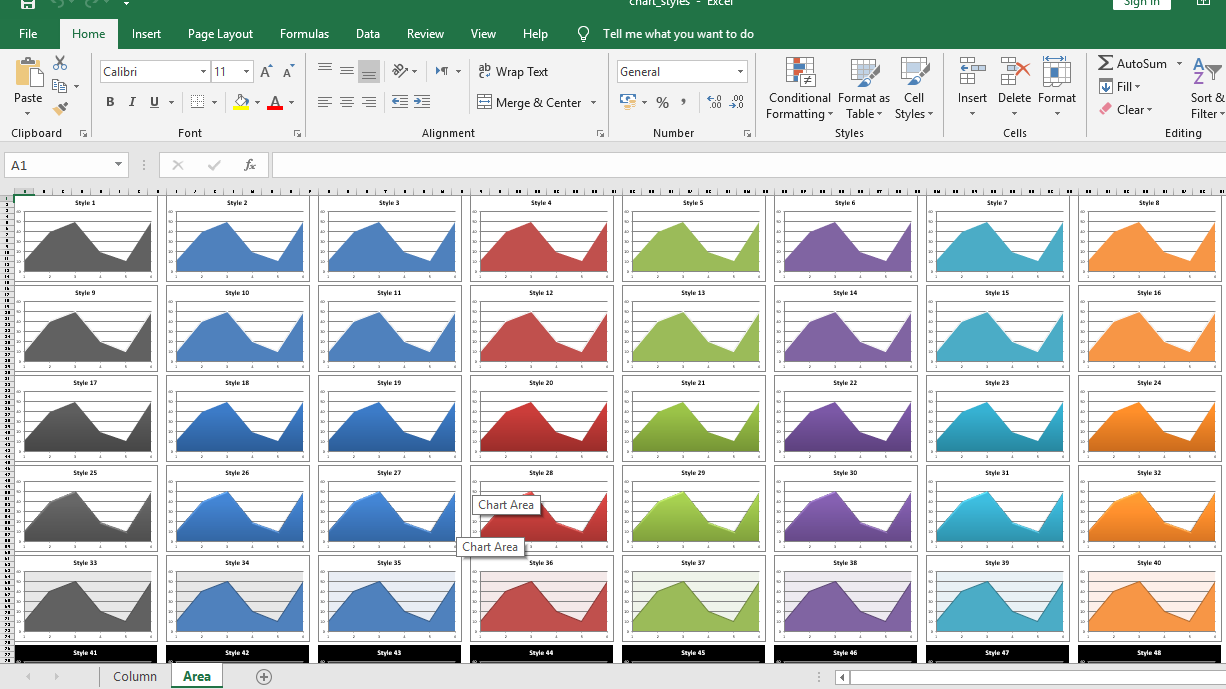# Python | Plotting Different types of style charts in excel sheet using XlsxWriter module

Prerequisite: Create and Write on an excel sheet

`XlsxWriter` is a Python library using which one can perform multiple operations on excel files like creating, writing, arithmetic operations and plotting graphs. Let’s see how to plot different types of Style charts, using realtime data.

Charts are composed of at least one series of one or more data points. Series themselves are comprised of references to cell ranges. For plotting the charts on an excel sheet, firstly, create chart object of specific chart type( i.e Line, Column chart etc.). After creating chart objects, insert data in it and lastly, add that chart object in the sheet object.

Code : Plot different types of style charts.

For plotting different types of style charts on an excel sheet, `use set_style()`method of the chart object with respective style id .

 `# import xlsxwriter module  ` `import` `xlsxwriter ` ` `  `# Workbook() takes one, non-optional, argument    ` `# which is the filename that we want to create. ` `workbook ``=` `xlsxwriter.Workbook(``'chart_styles.xlsx'``) ` ` `  `# Show the styles for column and area chart types. ` `chart_types ``=` `[``'column'``, ``'area'``] ` ` `  `for` `chart_type ``in` `chart_types: ` `     `  `    ``# The workbook object is then used to add new    ` `    ``# worksheet via the add_worksheet() method. ` `    ``# Add a worksheet for each chart type ` `    ``worksheet ``=` `workbook.add_worksheet(chart_type.title()) ` ` `  `    ``# set zoom option ` `    ``worksheet.set_zoom(``30``) ` ` `  `    ``# initialize style ` `    ``style_number ``=` `1` ` `  `    ``# Create 48 built-in styles, each with a different style. ` `    ``# each chart dimension is 15 X 8. ` `    ``for` `row_num ``in` `range``(``0``, ``90``, ``15``): ` `        ``for` `col_num ``in` `range``(``0``, ``64``, ``8``): ` ` `  `            ``# Create a chart object that can be added  ` `            ``# to a worksheet using add_chart() method.  ` ` `  `            ``# here we create a respective chart object . ` `            ``chart ``=` `workbook.add_chart({``'type'``: chart_type}) ` `             `  `            ``# Add a data series to a chart  ` `            ``# using add_series method.  ` `            ``chart.add_series({``'values'``: ``'= Data !\$A\$1:\$A\$6'``}) ` ` `  `            ``# Add a chart title   ` `            ``chart.set_title ({``'name'``: ``'Style % d'` `%` `style_number}) ` ` `  `            ``# Turn off the chart legend. ` `            ``chart.set_legend({``'none'``: ``True``}) ` ` `  `            ``# Set an Excel chart style. ` `            ``chart.set_style(style_number) ` ` `  `            ``# add chart to the worksheet  ` `            ``# at the top-left corner of ` `            ``# a chart is anchored to ` `            ``# respective position of cell.   ` `            ``worksheet.insert_chart(row_num, col_num, chart) ` ` `  `            ``# do increment ` `            ``style_number ``+``=` `1` ` `  `# The workbook object is then used to add new    ` `# worksheet via the add_worksheet() method.  ` `# create a worksheet for writing data.  ` `data_worksheet ``=` `workbook.add_worksheet(``'Data'``) ` ` `  `# create a data list .  ` `data ``=` `[``10``, ``40``, ``50``, ``20``, ``10``, ``50``] ` ` `  `# Write a column of data starting from 'A1' ` `data_worksheet.write_column(``'A1'``, data) ` ` `  `# hide the data worksheet ` `data_worksheet.hide() ` ` `  `# Finally, close the Excel file   ` `# via the close() method.   ` `workbook.close() `

Output :My Personal Notes arrow_drop_upCheck out this Author's contributed articles.

If you like GeeksforGeeks and would like to contribute, you can also write an article using contribute.geeksforgeeks.org or mail your article to contribute@geeksforgeeks.org. See your article appearing on the GeeksforGeeks main page and help other Geeks.

Please Improve this article if you find anything incorrect by clicking on the "Improve Article" button below.

Article Tags :

28

Please write to us at contribute@geeksforgeeks.org to report any issue with the above content.